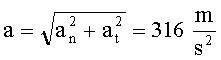# What is circular motion and its examples

## Trajectory

Circular motion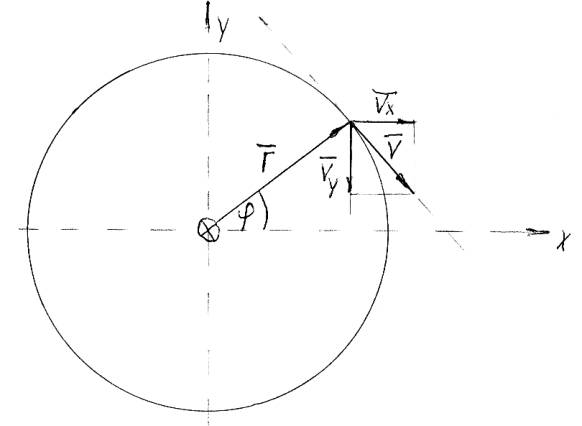Cartesian coordinates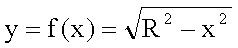Polar coordinates radius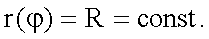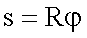Track length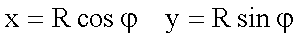### Path (tangential) speed

 Cartesian coordinates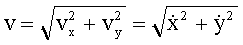Polar coordinates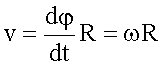### Angular velocity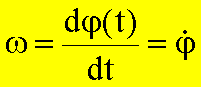The vector of the angular velocity is directed parallel to the axis of rotation (axial vector) and perpendicular to the plane of the path. It is then perpendicular to the radius vector and perpendicular to the vector of the tangential velocity. Its direction can be clearly described by the direction of movement of a screw with a right-hand thread. The right thumb rule applies analogously.The angular velocity w (angular difference per unit of time) is also called the angular frequency. You can from the frequency f (revolutions per unit of time) by means of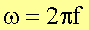be calculated.

The relationship between angular velocity, path velocity and radius vector is described by the Euler equation:

### Euler equation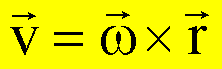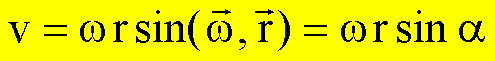#### Discussion of the Euler equationThe acceleration is obtained from the first derivative of the speed with respect to time: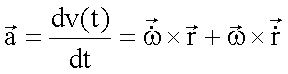Inserting the owler relationship for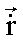results for the total or linear acceleration: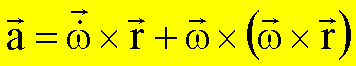The individual sizes have the following meaning:

 Angular acceleration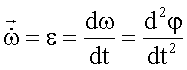Tangential orAzimuthal acceleration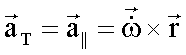Normal, radial orCentripetal acceleration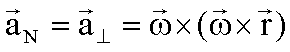Total acceleration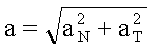The equations above apply under the assumption that a mass point is at rest in the rotating frame of reference.

The following graphic shows the individual components of the acceleration: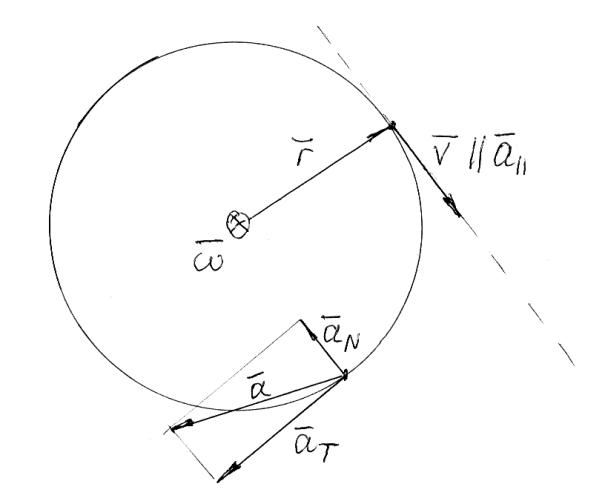The amount of the total acceleration results from this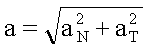Also for aT = 0 the normal acceleration is not equal to zero. The circular movement is therefore always an accelerated movement.

Circular movement with tangential acceleration = 0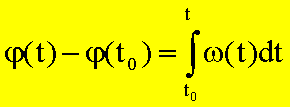Because of vT = const. And w (t) = w = const. follows: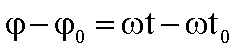The following applies to a full cycle: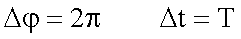and thus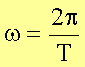or with the frequency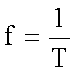also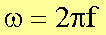The circular motion with constant path velocity v = wR is an accelerated motion. In order to maintain the circular motion, a force directed towards the center must be applied - the centripetal force. The following applies to the amount of centripetal force (see above):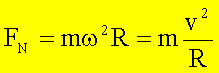Since the direction of the force changes continuously, the circular motion is an unevenly accelerated motion.

The circular motion with constant

Tangential acceleration

If one speaks of the uniformly accelerated circular movement, this means a circular movement with constant angular acceleration. As already discussed above, the uniform circular motion is already accelerated unevenly.

Outfollows with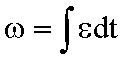the angular velocity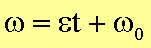Furthermore you get with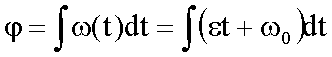###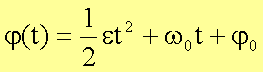The radial speed is equal to zero, the radial acceleration is equal to the centripetal acceleration.

### Example hammer thrower

A mass of m = 7.2 kg is accelerated uniformly on a radius of R = 2m and released at the angle j = 45 ° (maximum range) to the vertical. The maximum frequency of rotation of fMax = 2s-1 is reached after n = 3 revolutions.

·       Orbital speed and throwing distance

The maximum current path speed is reached after 3 revolutions at the end of the acceleration phase: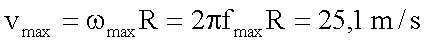The achievable throw is thus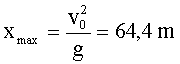·       Normal acceleration and centripetal force

##### The maximum radial acceleration follows from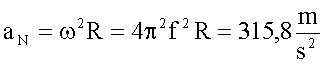The maximum normal force component to be used to keep the mass on the circular path is thus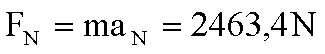·       Angular, path and total acceleration

Requirement: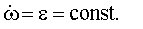Þ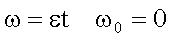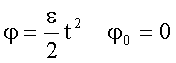If one eliminates t from the last two equations, it follows:At the end of the acceleration phase you get with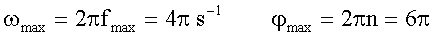an angular acceleration of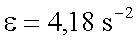This results in a tangential acceleration of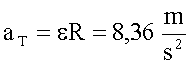This results in an overall acceleration of